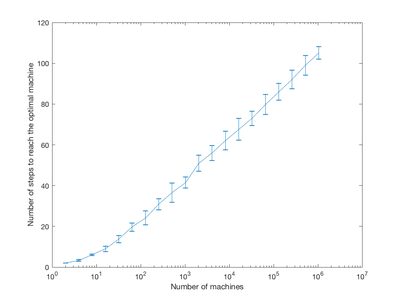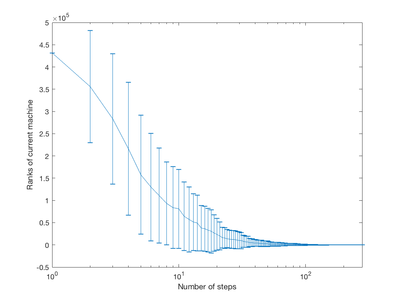# Course:CPSC522/Baseilne of RSI

Jump to navigation Jump to search

## A Theoretical Baseline of Recursive Self-improvement

This page formulates a family of RSI system, and analyzes the time complexity of simplified systems empirically.

Principal Author: Wenyi Wang
Collaborators:

## Abstract

Recursive self-improving systems have been dreamed since the early days of computer science and artificial intelligence. However current research on this topic remains vague and lacks clear formulation. In this page, we formulate a class of recursive self-improving (RSI) systems. For a more restricted class of RSI systems, we show that one of the RSI systems satisfies certain consistency, and it is computable. We study empirically that the RSI system we derived has $log(n)$runtime complexity where $n$is the size of search space for the best program.

### Builds on

This page studies a specific class of RSI systems. The algorithm described can also be viewed as stochastic optimization.

### Related Pages

The result of this work supports the possibility of intelligence explosion and artificial general intelligence.

## Content

### Introduction

Recursive self-improving systems create new software iteratively. The newly created software should be better at creating future software. With this property, the system has potential to completely rewrite its original implementation, and take completely different approaches . Chalmers' proportionality thesis hypothesizes that increases in the capability of designing future intelligent systems are propositional to the increases in intelligence. With this hypothesis, he shows if a process iteratively generates a greater intelligent system using the current system, this process leads to superintelligence . However current studies of RSI systems lack clear mathematical formulation of the object of interest (i.e. the RSI system). This work is motivated to overcome this weakness by formulating a class of RSI procedures. With this formulation, we show that one such RSI system is computable. We further study empirically that this procedure takes logarithmic runtime with respect to the size of search space to find the best program.

### The Mathematical Formulation for A Family of RSI Systems

In this section, we develop a mathematical formulation for a family of RSI systems. To develop the formulation, we need to exam the elements of an RSI system. An RSI system iteratively improves its current program on the ability to improve a future program. In this sentence, there are crucial two concepts being considered. First, an RSI system can be considered as a sequence of programs where each program in the sequence generates the next program. Second, each program in the sequence has (monotonically or asymptotically) increasing ability to improve future programs. Therefore, to define an RSI procedure a set of programs that can generate programs and an order of programs' ability to improve future programs are needed. In the following of this paper, we consider a finite search space of programs that generate programs and a total order over it. Notice that a total order over a finite set is isomorphic to a score function. Denote the set of programs by $P$and the score function by $S$. For convenience, let a lower score represent a higher order. Then an RSI system can be described as following.

Fix a finite set of programs $P$that generate programs and a score function $S$over $P$. Initialize $p$from $P$to be the system's current program. Repeat until certain criterion satisfied, generate $p'\in P$using $p$. If $p'$is better than $p$according to S, replace $p$by $p'$.

One unclarity remains that how a program $p\in P$generate a program? In general, we should allow them to generate programs based on previous histories of the entire process. In the following of this paper, we will assume a simplification that all the programs generated by the same program follow i.i.d. distributions. In another word, the way a program generates program is independent of the history, and each program defines a fixed probabilistic distribution over $P$. This procedure defines a stationary Markov chain. We will see that even with this restriction, with some score function, the model is able to achieve desired runtime performance.

### The Score Function as Expected Number of Steps

The last section defines an RSI procedure given a finite set of programs and a score function over it. We have specified the programs, but not the score function. Recall that the score function is to measure the programs' ability of future improvement. Consider when there is an optimal program that we want to find. The problem of finding a subset of the programs can be reduced to the same form by considering the target subset as a single element. The expected numbers of programs to generate starting from a program to find the optimal program following the defined procedure is a reasonable choice to describe the program's ability of future improvement. Furthermore, the score function needs to be consistent with the expected numbers of steps from programs to the optimal program following the process defined by itself. By consistency we mean that a score function $S$is consistent if for all $p,p'\in P$, $S(p)>S(p')$implies that the expected number of programs to generate starting from $p$is greater than starting from $p'$. More generally, if one takes some measure for programs' ability of future improvement based on the behaviour of the previously defined RSI procedure by a score function, then the score function needs to be consistent with this measure. The following of this section will show that there is a computable score function that is consistent with the expected numbers of steps.

Construct the score function as the expected numbers of steps to reach the optimal program by iteratively expanding the Markov chain for corresponding RSI procedure in a increasing order of scores. The intermediate Markov chains always follow the rule of transition defined by program distributions and current scores. It is obvious that the optimal program should have the minimum score (smaller score represents more preferred program). Initially add the optimal program to the Markov chain, and set its score equals zeros. Then repeat until all programs are added to the Markov chain. At each step, add program $p$to the Markov chain where $p$has the minimum expected number of steps to reach the optimal program if add it to the Markov chain. Then update the score of $p$as the expected number of steps to reach the optimal program in current Markov chain. This process of computing the score function can be done in $O(nlogn+m)$time in a similar way as the Dijkstra algorithm where $n$is the size of programs, and $m$is the sum of the number of possible programs that each program can generate.

Few nice properties hold for this construction. First, the score function equals the expected numbers of steps to reach the optimal program defined by this score function. Second, the programs are added in increasing order of scores.

### Experimental ResultsFigure 1: Expected number of steps from the first program to optimal program for different size of program set.Figure 2: Rank of the first program to optimal program for different size of program set.Caption: Figure 3: Simulation results of ranks of program at different step numbers for program set of size $2^{20}$We test the performance of the proposed RSI procedure in simulation with randomly generated abstraction of programs. For each of the experiments, a fixed number of programs is chosen from $n=2^{l},l=1,2,\dots ,20$. The first program is designed to generate programs uniformly over all programs. Other programs generate programs follow a weighted distribution over a subset of programs. The sizes of subsets are drawn i.i.d. from the uniform distribution over integers between 10 and 100. Given the size of a subset, the subset and corresponding weights are drawn uniformly over the feasible supports. With 10 repeats for $l=1,2,\dots ,20$, the expected number of steps for the first program to reach the optimal program and its rank over all programs are shown in figure 1 and 2. The figures suggest linear relation between $l$and expected number of steps and between $n$and rank of the first program. A linear regression model fits $l$and expected number of steps returns an R-squared value equals 0.983, which indicates the linear model can explain a lot of this relation. Similarly, the linear regression fit to $n$and rank of the first program has R-squared value equals 1.0.

For a fixed RSI system with $n=2^{20}$, we run 100 simulations of proposed procedure starting from the first program. Figure 3 shows an error-bar of the ranks of current program at different number of steps of the simulation. We see that before some of the processes reach the optimal program, the ranks improve exponentially in the statistical sense. All of the processes converge to the global optimal program.

### Conclusion

In summary, we formulate a family of RSI procedures. For a more restricted family of RSI procedures, we prove that a consistent score function exists, and we describe an algorithm to compute it. We study runtime of the restricted systems empirically. Experimental results suggest a logarithmic relation between the runtime and the number of programs. These results indicate a possibility of recursive self-improvement. For the future works, we have an intuition that the consistent score function might be optimal and unique. We could expand the model by embedding histories when generating a new program. Another possible extension is to model the programs taking an argument as a program and return a suggested improvement of the given program. From the practical point of view, to make the proposed procedure applicable, one needs to design an evaluable score function. One possible approach is to let each program take an argument as different program design tasks that can be evaluated, and evaluate a program based on its performance on the evaluable tasks. Since the practical score functions may not have the desired properties as we analyzed in the ideal case, it would be interesting to study the behaviour of proposed procedures when the score function is biased, noised or inconsistent.

## Annotated Bibliography

1. Yampolskiy, R. V. (2015). From seed AI to technological singularity via recursively self-improving software. arXiv preprint arXiv:1502.06512.
2. Chalmers, D. J. (2010). The singularity. Science Fiction and Philosophy: From Time Travel to Superintelligence, Second Edition, 171-224.

## To Add

Put links and content here to be added. This does not need to be organized, and will not be graded as part of the page. If you find something that might be useful for a page, feel free to put it here.Permission is granted to copy, distribute and/or modify this document according to the terms in Creative Commons License, Attribution-NonCommercial-ShareAlike 3.0. The full text of this license may be found here: CC by-nc-sa 3.0